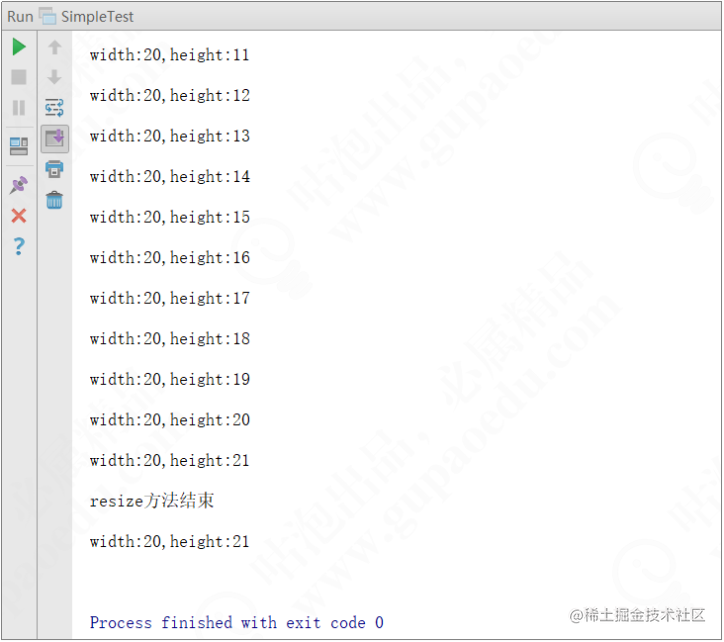# 软件架构设计原则之里氏替换原则

（1）子类可以实现父类的抽象方法，但不能覆盖父类的非抽象方法。

（2）子类可以增加自己特有的方法。

（3）当子类的方法重载父类的方法时，方法的前置条件（即方法的输入/入参）要比父类方法的输入参数更宽松。

（4）当子类的方法实现父类的方法时（重写/重载或实现抽象方法），方法的后置条件（即方法的输出/返回值）要比父类更严格或与父类一样。

``````public class JavaDiscountCourse extends JavaCourse {

public JavaDiscountCourse(Integer id, String name, Double price) {

super(id, name, price);

}

public Double getDiscountPrice(){

return super.getPrice() * 0.61;

}

}

（1）约束继承泛滥，是开闭原则的一种体现。

（2）加强程序的健壮性，同时变更时也可以做到非常好的兼容性，提高程序的可维护性和扩展性，降低需求变更时引入的风险。

``````public class Rectangle {

private long height;

private long width;

@Override

public long getWidth() {

return width;

}

@Override

public long getLength() {

return length;

}

public void setLength(long length) {

this.length = length;

}

public void setWidth(long width) {

this.width = width;

}

}

``````public class Square extends Rectangle {

private long length;

public long getLength() {

return length;

}

public void setLength(long length) {

this.length = length;

}

@Override

public long getWidth() {

return getLength();

}

@Override

public long getHeight() {

return getLength();

}

@Override

public void setHeight(long height) {

setLength(height);

}

@Override

public void setWidth(long width) {

setLength(width);

}

}

``````public static void resize(Rectangle rectangle){

while (rectangle.getWidth() >= rectangle.getHeight()){

rectangle.setHeight(rectangle.getHeight() + 1);

System.out.println("width:"+rectangle.getWidth() + ",height:"+rectangle.getHeight());

}

System.out.println("resize方法结束" +

"\nwidth:"+rectangle.getWidth() + ",height:"+rectangle.getHeight());

}

``````public static void main(String[] args) {

Rectangle rectangle = new Rectangle();

rectangle.setWidth(20);

rectangle.setHeight(10);

resize(rectangle);

}``````public static void main(String[] args) {

Square square = new Square();

square.setLength(10);

resize(square);

}

``````public interface Quadrangle {

long getWidth();

long getHeight();

}

``````public class Rectangle implements Quadrangle {

private long height;

private long width;

@Override

public long getWidth() {

return width;

}

public long getHeight() {

return height;

}

public void setHeight(long height) {

this.height = height;

}

public void setWidth(long width) {

this.width = width;

}

}

``````public class Square implements Quadrangle {

private long length;

public long getLength() {

return length;

}

public void setLength(long length) {

this.length = length;

}

@Override

public long getWidth() {

return length;

}

@Override

public long getHeight() {

return length;

}

}

「欢迎在评论区讨论，掘金官方将在掘力星计划活动结束后，在评论区抽送100份掘金周边，抽奖详情见活动文章」